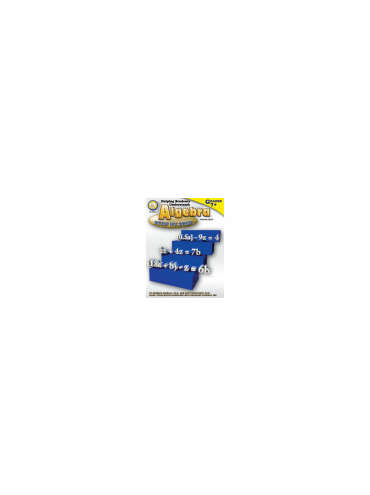••# Helping Students Understand Algebra Step By Step

Product Number: CD-404020
\$13.99
Facilitate a smooth transition from arithmetic to algebra for students in grades 7 and up using Helping Students Understand A...
Quantity

Facilitate a smooth transition from arithmetic to algebra for students in grades 7 and up using Helping Students Understand Algebra.

This 128-page math book includes step-by-step instructions with these resources:

• examples
• practice problems using the concepts
• real-life applications
• a list of symbols and terms
• tips

The book supports NCTM standards and includes chapters on these topics:

• number systems
• properties of numbers
• exponents and expressions
• algebraic expressions
• graphing
• functions

Features:

CD-404020

7
9